Bonky Zhu
If someone is able to show me that what I think or do is not right, I will happily change, for I seek the truth, by which no one was ever truly harmed. It is the person who continues in his self-deception and ignorance who is harmed.

# 编译原理 – 语法分析之自上而下的语法分析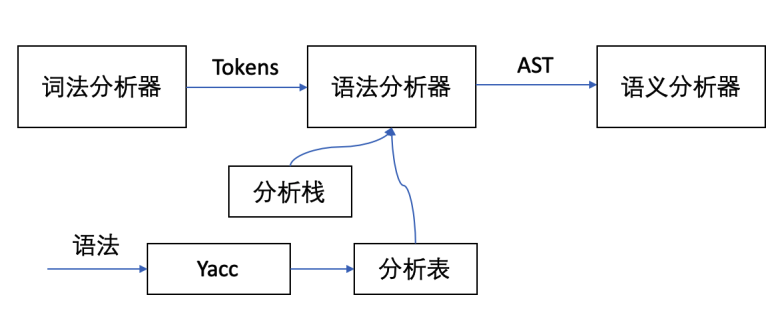## 基本概念

• 从推导的角度看，从开始符号出发，使用最左推导，试图推导出与输入符号串相同的句子。
• 从语法树的角度看，从根节点出发，反复使用所有可能的产生式，谋求输入串的匹配，试图向下构造一棵语法树，其末端节点正好与输入符号串相同

• 不确定的分析方法称为带回溯的分 析方法，这种方法实际上是一种穷举的试探方法
• 确定的分析方法不用回溯，需对文法有一定的限制，要求文法不能含有左递归,如LL(1)文法。

## 递归向下分析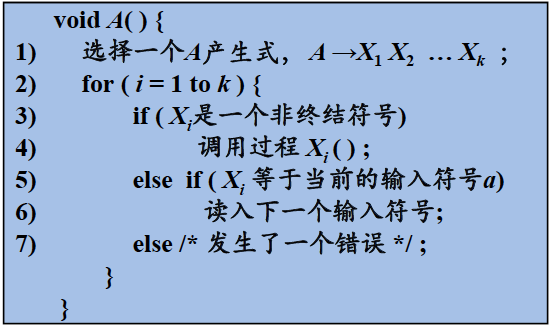PS：下面是我编译实验课所写的一个代码的框架，可以看到一共有 E A B C 四个终结符，每个终结符就和上面所说，对应了一个类似的函数：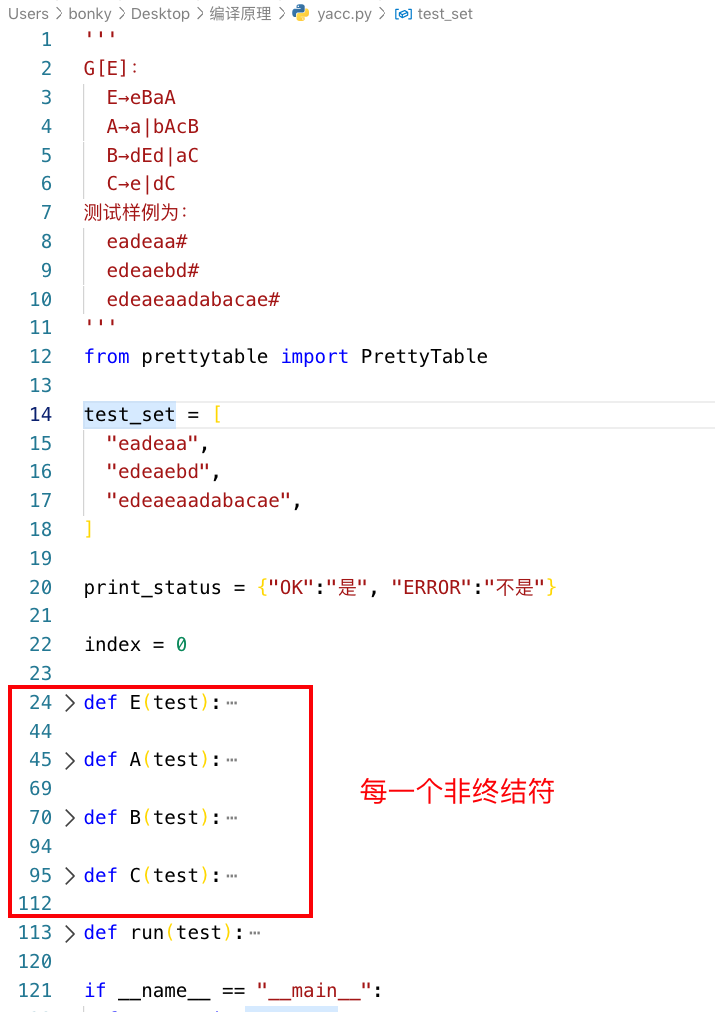### 左递归

E → E + T | E – T | T T → T * F | T / F | F F → (E) | id 

id + id * id ??  

### 消除简单左递归的方法

# 直接左递归 A → Aα|β  # 右递归 A → βA’  A’ → αA’|ε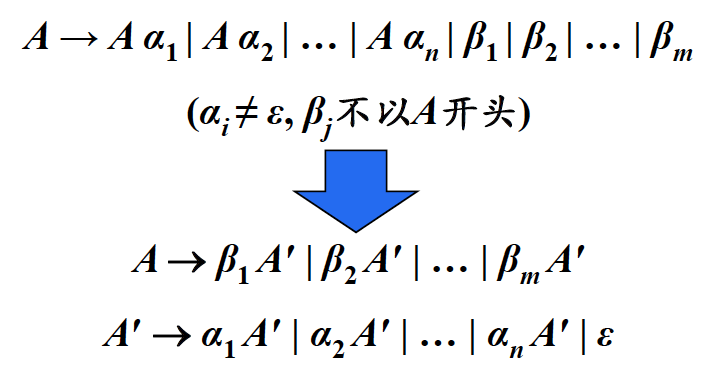### 消除间接左递归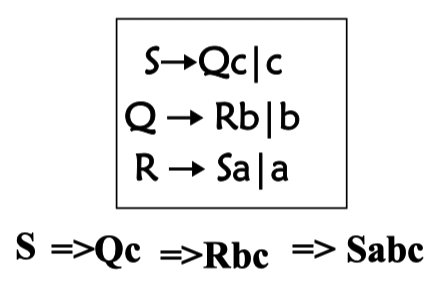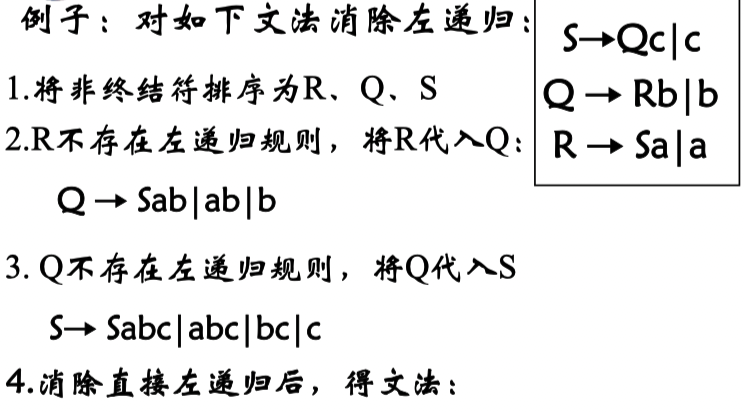### 提取左公共前缀

S → aAd | aBe 可以改写为 S → aS' 和 S' → Ad | Be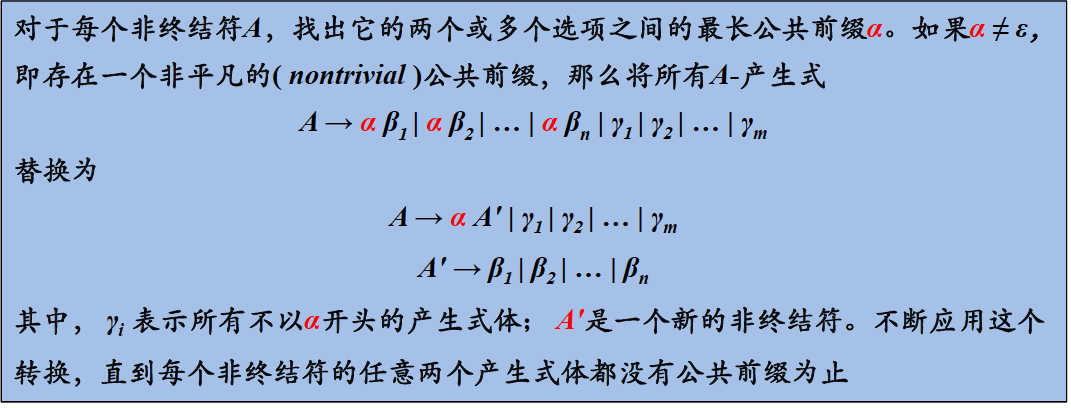## LL(1) 文法

### S_文法

• 每个产生式的右部都以终结符开始
• 同一非终结符的各个候选式的首终结符都不同

① S → aBC    ② B → bC     ③ B → dB  ④ B → ε ⑤ C → c    ⑥ C → a    ⑦ D → e 

### q_文法

• 每个产生式的右部为ε ，或者终结符开始
• 具有相同左部的产生式有不相交的SELECT集，如下面的就是没有相交的SELECT集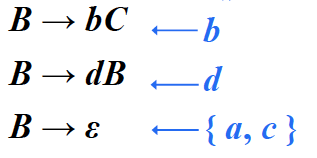### FIRST集

• 如果 ε 不属于 FIRST(α) , 那么 SELECT(A→α) = FIRST(α) （不可能选择为空产生式）
• 如果 ε 属于 FIRST(α), 那么 SELECT(A→α) = ( FIRST(α) - {ε} ) ∪ FOLLOW(A) （排除了产生空产生式的可能性）

### LL(1)文法

• 如果 α 和 β 均不能推导出 ε ，则 FIRST (α) ∩ FIRST (β) = Φ
• α 和 β 至多有一个能推导出 ε （如果两个都能，说明他们的 SELECT 集都包含 FOLLOW(A) ，SELECT集一定有相交）
• 如果 β 最终能推出 ε，则 FIRST(α) ∩ FOLLOW(A) = Φ，因为 β 的 SELECT 集包含 FOLLOW(A)，因此，α 的SELECT 集不包含 FOLLOW(A)，即交集为空。同理如果 α 最终能推出 ε，则 FIRST (β) ∩ FOLLOW(A) =Φ

## 语法分析的求解

### 文法符号FIRST集的求法

① E → TE' ② E' → +TE' | ε      ③ T → FT '  ④ T' → *FT' | ε  ⑤ F → (E) | id 

FIRST(E)  = {  } FIRST(E') = { + } FIRST(T)  = {  } FIRST(T') = { * } FIRST(F)  = { ( , id } 

FIRST(E)  = {  } FIRST(E') = { +, ε } FIRST(T)  = {  } FIRST(T') = { *, ε } FIRST(F)  = { ( , id } 

FIRST(E)  = { ( , id } FIRST(E') = { +, ε } FIRST(T)  = { ( , id } FIRST(T') = { *, ε } FIRST(F)  = { ( , id } 

### 串的FIRST 集的求法

• FIRST(X1, X2, … ,Xn) 加入FIRST(X1)中所有的非ε符号，如果 ε 在 FIRST(X1) 中，再加入 FIRST(X2) 中的所有非 ε 符号；
• 如果 ε 在 FIRST(X1)FIRST(X2) 中，再加入 FIRST(X3) 中的所有非ε符号，以此类推
• 最后，如果对所有的 i，ε 都在 FIRST(Xi) 中，那么将 ε 加入到 FIRST(X1，X2，…，Xn)

### 非终结符A的FOLLOW(A)

FIRST(E)  = { ( , id } FIRST(E') = { +, ε } FIRST(T)  = { ( , id } FIRST(T') = { *, ε } FIRST(F)  = { ( , id } 

• 取一条产生式，对产生式的每一个非终结符分析：
• 对于非最右边的非终结符，FOLLOW 集加上下一个的终结符，或者下一个非终结符的 FIRST 集减去 ε
• 如果下一个非终结符的 FIRST 集包括 ε，那么 FOLLOW 集再加上下一个非终结符的 FOLLOW
• 对于为最右边的非终结符，FOLLOW 集加上结束符 #，产生式左边的 FOLLOW 集等于最右边非终结符的 FOLLOW 集，如 E → TE' ，恒有 FOLLOW(E) = FOLLOW(E') （能跟在E后面的，展开之后肯定也能跟在 E' 后面）
• 继续向下取产生式，取到最底重新从头开始取产生式，直到各非终结符的 FOLLOW 集不在变化

### SELECT集

• 产生式右部第一个为终结符： SELECT 集就为这个终结符
• 产生式右部第一个为 ε： SELECT 集就为产生式左部的 FOLLOW
• 产生式右部第一个为非终结符：
• 如果第一个的 FIRST 集不存在 ε， SELECT 集等于第一个的 FIRST
• 如果第一个的 FIRST 集存在 ε， SELECT 集等于 (FIRST - {ε}) ∪ FOLLOW(A)

### 预测分析表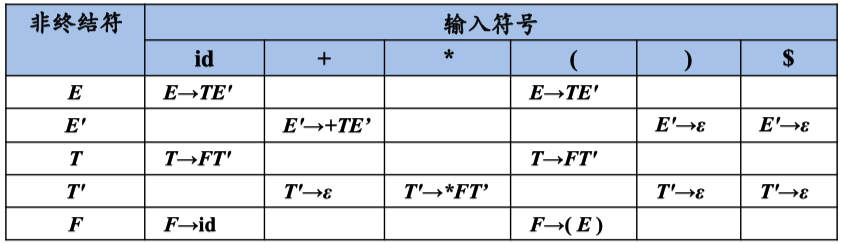Share